Trigonometric calculations (sine and tangent)

Trig scales on back of the slide

Introduction

It is not the purpose of this site to teach trigonometry, rather how to use trig scales on slide rules. For this purpose we will look at typical examples using trig scales in different positions on the rule.  This page is related to rules with the trig scales on the back of the slide with windows on the back of the rule.

As an example we use a Blundell 606. This 5" rule has three trig scales, one for tan (T : 5.6° to 45° ), one for sin (S : 5.6° to 90°) and one for sin and tan for small angles (ST : 0.56° to 5.6°). Not all rules have the ST scale. We discuss below how to calculate the tan of angles outside the range of the scales. The advantage of a 5" rule is that distances are smaller which makes for more compact diagrams.

This rule has the angles graduated in degrees and minutes, though this is only apparent for smaller angles. Other rules may have the angles graduated in degrees and decimal fractions of a degree or in grads.

Right angle triangles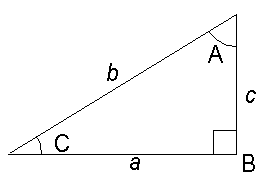Example 1
To find the length of c given the length of a (2.0) and angle C (30°).

The formula is:    c = a tan C

Method
T (bottom scale) to 30 on back of slide
Cursor to 2 on D
Answer (1.16) under cursor on C.

 Back view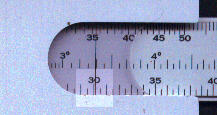Front view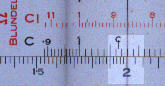Example 2
To find the angle C given the length of a (1.2) and b (2.3)

The formula is: C = sin-1 (a/b)

Method
Align 1.2 on C with 2.3 on D
Answer 31.4° under mark on S

 Front view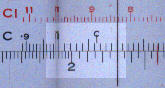Back view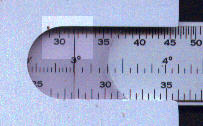Example 3

To find the length c given the angle C (27.0°) and the length b (1.8).

The formula is:
c = b sin C

Method
S (top scale) to 27.0 on back of slide
Cursor to 1.8 on D
Answer (0.817) under cursor on C.

 Back view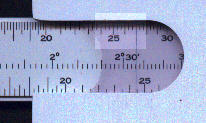Front view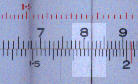Scalene triangles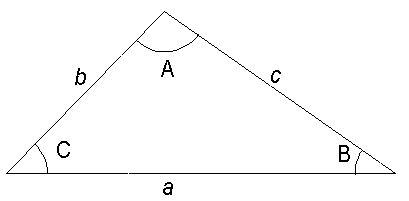Example 4
To find c given b (2.3) , C (47.0°) and B (32.0°)

The formula is:
c = b sin C / sin B

Method
S (top scale) to 32.0 on back of slide
Cursor to 2.3 on C.
S (top scale) to 47.0 on back of slide
Answer (3.17) under cursor on C.

 Back view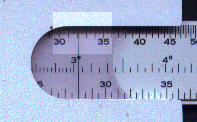Front view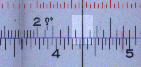Back view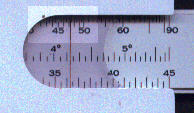Front view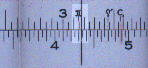Tan and sin of small angles (<5.7°)

For small angles tan and sin are almost equal. To calculate the sin or tan of a small angle multiply the angle by 0.01745. Some rules have a gauge point at this value.

Tan of large angles ( > 45.0°)

For slide rules without a second tan scale align 90 minus the angle on the tan scale with the mark on the back, the answer is on the D scale under 1 of the C scale.

The example shows tan 60.
90 - 60 = 30
30 on the tan scale is aligned with mark.
The answer 1.73 is on the D scale against 1 of the C scale.

 Back viewFront view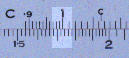Variants

One variant, which is quite common, is for the scales on the back of the slide to be used with the A scale of the front. As the A scale covers two log cycles this enables values of 0.56° to 90.0° degrees to be covered with a single scale but, of course, reduced accuracy.

A sub-variant of this arrangement is to have two marks on the slide as shown below.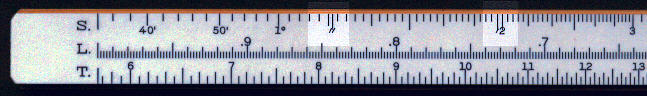Both of these marks are for finding the sine of very small angles (or tangents as for small angles sin and tan are equal). The one near to 2 degrees is for minutes and the one near to 1° 10' is for minutes.

Example 5
To find the sine of 10'.

Minute mark on slide to appropriate mark on window at the back of the rule
The answer (0.00291) is on the A scale opposite 10 in the B scale.

 Back view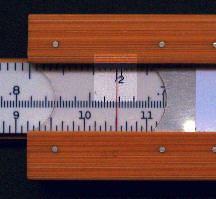Front view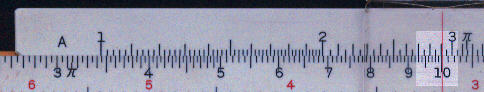A similar method is used for seconds.